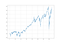# Using dark pools as predictors of equity market turning points.

## Peaking into dark pools for market forecasting.

Dark pools are private trading exchanges that are not available to outside retail investors. The main reason for the existence of dark pools is to facilitate block trading between big institutional investors. Knowing the movements of dark pool operators is a valuable add-on to equity trading. In this article, we will create two strategies using two different “behind-the-curtains” indicators in predicting market movements. Our aim is to see whether they are useful or not, especially that the two indicators are publicly available.The S&P500 since the beginning of May 2011 returned around 150.00%.

# Strategy #1: The Dark Index-DIX

The Dark index provides a way of peeking into the secret (dark) exchanges. it is calculated as an aggregate value of many dark pool indicators (another type of release provided by squeezemetrics) and measures the hidden market sentiment. When the values of this indicator are higher than usual, it means that more buying occured in darl pools than usual. We can profit from this informational gap. It is therefore a trail of liquidity providers (i.e. negatively correlated with the market it follows due to hedging activities).

`def normalizer(Data, norm_lookback, position, what_normalize):        for i in range(len(Data)):        try:                Data[i, position] = (Data[i, what_normalize] - min(Data[i - norm_lookback:i + 1, what_normalize])) \                / (max(Data[i - norm_lookback:i + 1, what_normalize]) - min(Data[i - norm_lookback:i + 1, what_normalize]))                        except ValueError:            pass`
• Short sell whenever the DIX normalized hits 0.00.
`Profit factor        =  2.515Hit ratio            =  50.59 %Expectancy           =  31.43`

# Strategy #2: The gamma exposure index -GEX

The gamma exposure index relates to the sensitivity of option contracts to changes in the underlying price. When imbalances occur, the effects of market makers’ hedges may cause price swings (such as short squeezes). The absolute value of the GEX index is simply the number of shares that will be bought or sold to push the price in the opposite direction of the trend when a 1% absolute move occurs. For example, if the price moves +1% with the GEX at 5.0 million, then, 5.0 million shares will come pouring in to push the market to the downside as a hedge. As documented, the GEX acts as a brake on prices when it’s high enough with a rising market.

• Short sell whenever the GEXX normalized hits 1.00.
`Profit factor        =  1.638Hit ratio            =  39.56 %Expectancy           =  14.93`
• Adding these two powerful sentiment indicators to the trading strategy can raise convictions.
• As the DIX is negatively correlated with the US market while the GEX is positively correlated, we can form a composite of the GEX-DIX difference that may even help give us better trading signals.

Written by